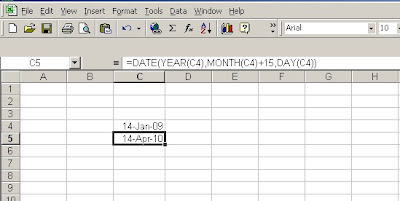## Sunday, January 4, 2009

### Excel date mathematics

Microsoft Excel stores dates as sequential numbers known as serial values. Excel stores times as decimal fractions because time is considered a portion of a day.

Dates and times are values and, therefore, can be added, subtracted, and included in other calculations. Will tell you date mathematics formula.

How to Add / Subtract month from a date in Excel.
Adding / Subtracting a month from a particular date in excel looks tough as each month has different number of days. However it is very simple if you use following formula.
=DATE(YEAR(C4),MONTH(C4)+1,DAY(C4))

In this base date is available at cell C4 and we have added 1 to month value to increase it by one month. Similarly you can subtract required number of months from base date. See picture below for further understanding of it.Addition of more than 12 months will result into addition of year. See picture below:-Addition of 15 months have resulted into increase of one year and 3 months.

This will work for Year addition / subtraction also.